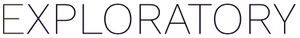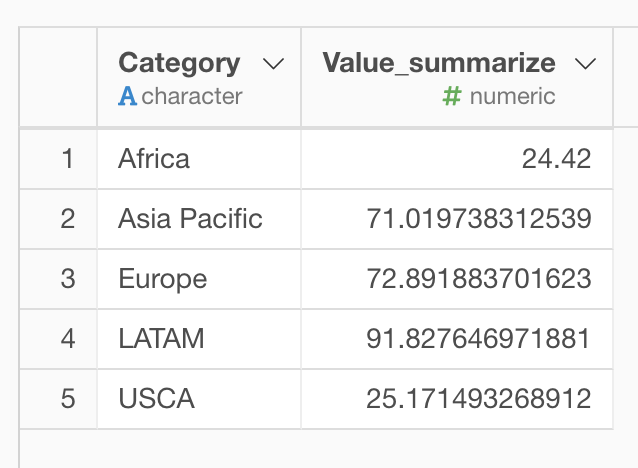# How to calculate geometric mean for each group?

#1

Suppose you have a data frame with “Category” and “Value” columns like following, and you want to calculate the geometric mean for each Category.

Here is how to calculate. First, you can “Group By” the “Category” Column from the column header menu.

Next, you can select “Summarize” -> “Custom” from the “Value” column header menu.

Then, type in like following.

``````prod(Value, na.rm=TRUE) ^ (1/n())
``````

This formula means 1) multiplying all the values by prod function, then 2) calculate the nth root. Since we did the “Group By” above, this calculation is done for each group.

Then you will get like this.If you have negative values in “Values” column, you may want to get rid of the negative by `abs` function before prod function.

2 Likes
#3

This approach has just come in handy for a related challenge, calculating skewness. I first installed the `moments` package.

#4

Here is a related post: How to calculate skewness of all the numeric columns in the data frame

1 Like